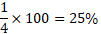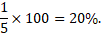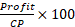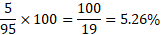• Save

Profit Loss Tricks for Competitive Exams

8 months ago 1.6K ViewsProfit and loss question takes time to solve. Students waste a lot of time while solving these questions in the competitive exam because they face difficulties. So, here I am sharing profit loss tricks for your better performance.

You can save your time in the competitive exams by learning these important tricks that how to solve profit loss questions in a short time. So, continue your practice with these questions to obtain good marks in the exam.

After learning tricks you can know Profit Loss Formulas if you have doubt any formula in which questions have been sorted out with formula.

Important Tricks of Profit and Loss:

Cost Price (CP): The money paid by the shopkeeper to the manufacturer or money paid by the customer to the shopkeeper is called the price (CP) of the goods.

Selling Price (SP): The price at which the shopkeeper sells the goods is called the selling price.

Here, I am providing important short tricks so you can easily solve profit and loss questions.

Useful Tricks:

(1) If SP is greater than CP then we have profit, so formula of profit will be =

SP>CP---- Profit

Profit = SP-CP

(2) If SP is Less than CP then we have Loss, so formula of Loss will be =

SP>CP-----Loss

Loss = CP-SP

(3) Profit and Loss is always counted on CP

(4) To solve any question of profit and loss, you have to always assume

CP = 100%

Q.1. A person incurs 5% loss by selling a watch for Rs. 1140. At what price should the watch be sold to earn 5% profit.

(A) 1320

(B) 1260

(C) 1210

(D) 1350

Ans .   B

Short Trick

CP = 100% {point no. 4, you have to always assume CP=100%)

If CP = 100% and Loss is 25% which mean SP= CP – L%

SP = 100% - 5% = 95%

So, we can say

SP = 95% = 1140

If CP=100% and Profit is 40% Which mean SP= CP+ Profit%

SP= 100%+5%=105%

So, we have to find value of 105% and 105% is equal to

= 1260.

So, New S.P = Rs. 1260.

Q.2. A Bought pens at the rate of 15 per rupee and sold them at the rate of 12 for a rupee. His gain percent is

(A) 25%

(B) 26%

(C) 50%

(D) 53%

Ans .   A

Shor Tricks

We are gating profit here, So

we have to assume bigger number will be SP and Smaller number will be CP.

We get ratio of   SP : CP

15  :  12

5   :   4

The difference of 5 : 4 is 1

Profit and loss always counted CP , which meansQ.3. If the cost price of 12 orange is equal to selling price of 10 orange. Then the profit or los percentage is :

(A) 10%

(B) 20%

(C) 30%

(D) 35%

Ans .  B

Short Trick

we have to assume bigger number will be SP and Smaller number will be CP.

We get ratio of   SP : CP

12 : 10

6 :  5

The difference of 6 : 5 is 1

Profit and loss always counted CP , which meansQ.4. A man sells an article at a profit of 40%. If he had bought it at 40% less and sold for Rs.5 less, he would have gained 50%. Find the cost price of the article.

(a) Rs. 10

(b) Rs. 15

(c) Rs. 20

(d) Rs. 30

Ans .  A

Short Trick

Let CP = RS. 100, Then SP = 140

New CP = Rs. 60, New SP = 60+30= Rs.90

According to the question.

Rs.(140-90)= Rs. 50 is equivalent to Rs. 5

⸫ Rs. 100 is equivalent to Rs. 10

⸫ CP = Rs. 10.

Q.5. If the cost price is 95% of the selling price, what is the profit percent?

(A) 4

(B) 4.75

(C) 5

(D) 5.26

Ans .  D

Short Trick

Let SP = Rs. 100

⸫ CP = Rs. 95.

Profit = Rs. (100-95)= Rs. 5

⸫ Profit percent ==You can ask me anything related profit loss tricks in the comment section without any hesitation.